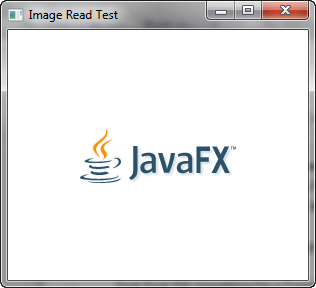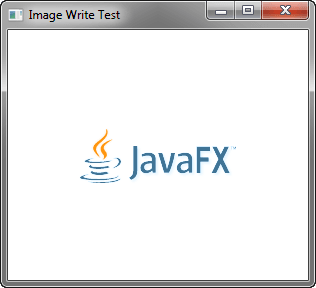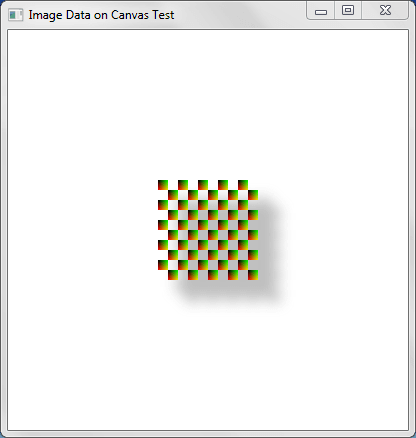Documentation

JavaFX: Working with JavaFX Graphics

# 10 Using the Image Ops API

This chapter introduces you to Image Ops, an API that enables you to read and write raw pixels within your JavaFX applications.

You will learn how to read pixel from images, write pixels to images, and create snapshots.

## Overview of the Image Ops API

The Image Ops API consists of the following classes/interfaces in the `javafx.scene.image` package:

• `Image`: Represents a graphical image. This class provides a `PixelReader` for reading pixels directly from an image.

• `WritableImage`: A subclass of `Image`. This class provides a `PixelWriter` for writing pixels directly to an image. A `WritableImage` is initially created empty (transparent) until you write pixels to it.

• `PixelReader`: Interface that defines methods for retrieving pixel data from an Image or other surface that contains pixels.

• `PixelWriter`: Interface that defines methods for writing pixel data to a `WritableImage` or other surface that contains writable pixels.

• `PixelFormat`: Defines the layout of data for a pixel of a given format.

• `WritablePixelFormat`: A subclass of `PixelFormat`, representing a pixel format that can store full colors. It can be used as a destination format to write pixel data from an arbitrary image.

The following sections demonstrate this API with examples that you can compile and run.

You may already be familiar with the `javafx.scene.image.Image` class, which (along with `ImageView`) is used in JavaFX applications that display images. The following example demonstrates how to display an image by loading the JavaFX logo from oracle.com and adding it to the JavaFX scene graph.

```package imageopstest;

import javafx.application.Application;
import javafx.scene.Scene;
import javafx.scene.layout.StackPane;
import javafx.stage.Stage;
import javafx.scene.image.Image;
import javafx.scene.image.ImageView;

public class ImageOpsTest extends Application {

@Override
public void start(Stage primaryStage) {

// Create Image and ImageView objects
Image image = new Image("http://docs.oracle.com/javafx/"
+ "javafx/images/javafx-documentation.png");
ImageView imageView = new ImageView();
imageView.setImage(image);

// Display image on screen
StackPane root = new StackPane();
Scene scene = new Scene(root, 300, 250);
primaryStage.setScene(scene);
primaryStage.show();
}

public static void main(String[] args) {
launch(args);
}
}
```

Running this program will produce the image shown in Figure 10-1.

Figure 10-1 Displaying an ImageDescription of "Figure 10-1 Displaying an Image"

Now, let's modify this code to read `Color` information directly from the pixels. You can do this by invoking the `getPixelReader()` method, and then using the `getColor(x,y)` method of the returned `PixelReader` object to obtain the pixel's color at the specified coordinates.

Example 10-2 Reading Color Information from Pixels

```package imageopstest;

import javafx.application.Application;
import javafx.scene.Scene;
import javafx.scene.layout.StackPane;
import javafx.stage.Stage;
import javafx.scene.image.Image;
import javafx.scene.image.ImageView;
import javafx.scene.paint.Color;

public class ImageOpsTest extends Application {

@Override
public void start(Stage primaryStage) {

// Create Image and ImageView objects
Image image = new Image("http://docs.oracle.com/javafx/"
+ "javafx/images/javafx-documentation.png");
ImageView imageView = new ImageView();
imageView.setImage(image);

System.out.println("Image Width: "+image.getWidth());
System.out.println("Image Height: "+image.getHeight());

// Determine the color of each pixel in the image
System.out.println("\nPixel color at coordinates ("
+ color.toString());
System.out.println("R = " + color.getRed());
System.out.println("G = " + color.getGreen());
System.out.println("B = " + color.getBlue());
System.out.println("Opacity = " + color.getOpacity());
System.out.println("Saturation = " + color.getSaturation());
}
}

// Display image on screen
StackPane root = new StackPane();
Scene scene = new Scene(root, 300, 250);
primaryStage.setScene(scene);
primaryStage.show();
}

public static void main(String[] args) {
launch(args);
}
}
```

This version uses nested for loops (that invoke the `getColor` method) to obtain color information from every pixel in the image. It reads in pixels one at a time, starting in the upper left corner (0,0) and progressing across the image from left to right. The Y coordinate increments only after an entire row has been read. Information about each pixel (color values, opacity and saturation values etc.) is then printed to standard output, proving that the read operations are working correctly.

`.``.. // beginning of output omitted`

`Pixel color at` `coordinates (117,27) 0x95a7b4ff`

`R = 0.5843137502670288`

`G = 0.6549019813537598`

`B = 0.7058823704719543`

`Opacity = 1.0`

`Saturation = 0.17222220767979304`

`Pixel color at coordinates (118,27) 0x2d5169ff`

`R = 0.1764705926179886`

`G = 0.3176470696926117`

`B = 0.4117647111415863`

`Opacity = 1.0`

`Saturation = 0.5714285662587809`

`... // remainder of output omitted`

You may be tempted to try modifying the color of each pixel and writing that to the screen. But keep in mind that Image objects are read-only; to write new data, you need an instance of `WritableImage` instead.

## Writing Pixels to Images

Now let's modify this demo to brighten each pixel, then write the modified result to a `WritableImage` object.

Example 10-3 Writing to a WritableImage

```package imageopstest;

import javafx.application.Application;
import javafx.scene.Scene;
import javafx.scene.layout.StackPane;
import javafx.stage.Stage;
import javafx.scene.image.Image;
import javafx.scene.image.ImageView;
import javafx.scene.image.PixelWriter;
import javafx.scene.paint.Color;
import javafx.scene.image.WritableImage;

public class ImageOpsTest extends Application {

@Override
public void start(Stage primaryStage) {

// Create Image and ImageView objects
Image image = new Image("http://docs.oracle.com/javafx/"
+ "javafx/images/javafx-documentation.png");
ImageView imageView = new ImageView();
imageView.setImage(image);

System.out.println("Image Width: "+image.getWidth());
System.out.println("Image Height: "+image.getHeight());

// Create WritableImage
WritableImage wImage = new WritableImage(
(int)image.getWidth(),
(int)image.getHeight());
PixelWriter pixelWriter = wImage.getPixelWriter();

// Determine the color of each pixel in a specified row
System.out.println("\nPixel color at coordinates ("+
+color.toString());
System.out.println("R = "+color.getRed());
System.out.println("G = "+color.getGreen());
System.out.println("B = "+color.getBlue());
System.out.println("Opacity = "+color.getOpacity());
System.out.println("Saturation = "+color.getSaturation());

// Now write a brighter color to the PixelWriter.
color = color.brighter();
}
}

// Display image on screen
imageView.setImage(wImage);
StackPane root = new StackPane();
Scene scene = new Scene(root, 300, 250);
primaryStage.setTitle("Image Write Test");
primaryStage.setScene(scene);
primaryStage.show();
}

public static void main(String[] args) {
launch(args);
}
}
```

This version creates a `WritableImage` initialized to the same width and height as the JavaFX logo. After obtaining a `PixelWriter` (for writing pixel data to the new image), the code invokes the `brighter()` method (to lighten the shade of the current pixel's color), then writes the data to the new image by invoking `pixelWriter.setColor(readX,readY,Color)`.

Figure 10-2 shows the result of this process.

Figure 10-2 A Brighter Logo, Stored in a WritableImage ObjectDescription of "Figure 10-2 A Brighter Logo, Stored in a WritableImage Object"

## Writing Images with Byte Arrays and PixelFormats

The demos so far have successfully obtained and modified pixel colors, but the code was still relatively simple (and not necessarily optimal), compared to what the API is capable of. Example 10-4 creates a new demo that writes pixels a rectangle at a time, using a `PixelFormat` to specify how the pixel data is stored. This version also displays the image data on a `Canvas`, instead of an `ImageView`. (See Working with the Canvas API for more information about the `Canvas` class.)

Example 10-4 Writing Rectangles to a Canvas

```package imageopstest;

import java.nio.ByteBuffer;
import javafx.application.Application;
import javafx.scene.Group;
import javafx.scene.Scene;
import javafx.scene.canvas.Canvas;
import javafx.scene.canvas.GraphicsContext;
import javafx.scene.image.PixelFormat;
import javafx.scene.image.PixelWriter;
import javafx.scene.paint.Color;
import javafx.stage.Stage;

public class ImageOpsTest extends Application {

// Image Data
private static final int IMAGE_WIDTH = 10;
private static final int IMAGE_HEIGHT = 10;
private byte imageData[] =
new byte[IMAGE_WIDTH * IMAGE_HEIGHT * 3];

// Drawing Surface (Canvas)
private GraphicsContext gc;
private Canvas canvas;
private Group root;

public static void main(String[] args) {
launch(args);
}

@Override
public void start(Stage primaryStage) {
primaryStage.setTitle("PixelWriter Test");
root = new Group();
canvas = new Canvas(200, 200);
canvas.setTranslateX(100);
canvas.setTranslateY(100);
gc = canvas.getGraphicsContext2D();
createImageData();
drawImageData();
primaryStage.setScene(new Scene(root, 400, 400));
primaryStage.show();

}

private void createImageData() {
int i = 0;
for (int y = 0; y < IMAGE_HEIGHT; y++) {
int r = y * 255 / IMAGE_HEIGHT;
for (int x = 0; x < IMAGE_WIDTH; x++) {
int g = x * 255 / IMAGE_WIDTH;
imageData[i] = (byte) r;
imageData[i + 1] = (byte) g;
i += 3;
}
}
}

private void drawImageData() {
boolean on = true;
PixelWriter pixelWriter = gc.getPixelWriter();
PixelFormat<ByteBuffer> pixelFormat = PixelFormat.getByteRgbInstance();
for (int y = 50; y < 150; y += IMAGE_HEIGHT) {
for (int x = 50; x < 150; x += IMAGE_WIDTH) {
if (on) {
pixelWriter.setPixels(x, y, IMAGE_WIDTH,
IMAGE_HEIGHT, pixelFormat, imageData,
0, IMAGE_WIDTH * 3);
}
on = !on;
}
on = !on;
}

}
}
```

Figure 10-3 Writing Pixels to a CanvasDescription of "Figure 10-3 Writing Pixels to a Canvas"

This demo does not read data from an existing image; it creates a new `WritableImage` object entirely from scratch. It draws several rows of multi-colored 10x10 rectangles, the color data for which is stored in an array of bytes representing the RGB values of each pixel.

Of particular interest are the private methods `createImageData` and `drawImageData`. The `createImageData` method sets the RGB values for the colors that appear in each 10x10 rectangle:

Example 10-5 Setting the RGB Values for Pixels

```...
private void createImageData() {
int i = 0;
for (int y = 0; y < IMAGE_HEIGHT; y++) {
System.out.println("y: "+y);
int r = y * 255 / IMAGE_HEIGHT;
for (int x = 0; x < IMAGE_WIDTH; x++) {
System.out.println("\tx: "+x);
int g = x * 255 / IMAGE_WIDTH;
imageData[i] = (byte) r;
imageData[i + 1] = (byte) g;
System.out.println("\t\tR: "+(byte)r);
System.out.println("\t\tG: "+(byte)g);
i += 3;
}
}
}
...
```

This method sets the R and G values for each pixel of the rectangle (B is always 0). These values are stored in the `imageData` byte array, which holds a total of 300 individual bytes. (There are 100 pixels in each 10x10 rectangle, and each pixel has R, G, and B values, resulting in 300 bytes total).

With this data in place, the `drawImageData` method then renders the pixels of each rectangle to the screen:

Example 10-6 Rendering the Pixels

```private void drawImageData() {
boolean on = true;
PixelWriter pixelWriter = gc.getPixelWriter();
PixelFormat<ByteBuffer> pixelFormat = PixelFormat.getByteRgbInstance();
for (int y = 50; y < 150; y += IMAGE_HEIGHT) {
for (int x = 50; x < 150; x += IMAGE_WIDTH) {
if (on) {
pixelWriter.setPixels(x, y, IMAGE_WIDTH,
IMAGE_HEIGHT, pixelFormat, imageData, 0, IMAGE_WIDTH * 3);
}
on = !on;
}
on = !on;
}
}
```

Here, the `PixelWriter` is obtained from the `Canvas`, and a new `PixelFormat` is instantiated, specifying that the byte array represents RGB values. The pixels are then written an entire rectangle at a time by passing this data to the `PixelWriter`'s `setPixels` method.

## Creating a Snapshot

The `javafx.scene.Scene` class also provides a snapshot method that returns a `WritableImage` of everything currently shown in your application's scene. When used in conjunction with Java's `ImageIO` class, you can save the snapshot to the filesystem.

Example 10-7 Creating and Saving a Snapshot

```package imageopstest;

import java.io.File;
import java.io.IOException;
import java.nio.ByteBuffer;
import javafx.application.Application;
import javafx.embed.swing.SwingFXUtils;
import javafx.scene.Group;
import javafx.scene.Scene;
import javafx.scene.canvas.Canvas;
import javafx.scene.canvas.GraphicsContext;
import javafx.scene.image.PixelFormat;
import javafx.scene.image.PixelWriter;
import javafx.scene.image.WritableImage;
import javafx.scene.paint.Color;
import javafx.stage.Stage;
import javax.imageio.ImageIO;

public class ImageOpsTest extends Application {

// Image Data
private static final int IMAGE_WIDTH = 10;
private static final int IMAGE_HEIGHT = 10;
private byte imageData[] = new byte[IMAGE_WIDTH * IMAGE_HEIGHT * 3];
// Drawing Surface (Canvas)
private GraphicsContext gc;
private Canvas canvas;
private Group root;

public static void main(String[] args) {
launch(args);
}

@Override
public void start(Stage primaryStage) {
primaryStage.setTitle("PixelWriter Test");
root = new Group();
canvas = new Canvas(200, 200);
canvas.setTranslateX(100);
canvas.setTranslateY(100);
gc = canvas.getGraphicsContext2D();
createImageData();
drawImageData();

Scene scene = new Scene(root, 400, 400);
primaryStage.setScene(scene);
primaryStage.show();

//Take snapshot of the scene
WritableImage writableImage = scene.snapshot(null);

// Write snapshot to file system as a .png image
File outFile = new File("imageops-snapshot.png");
try {
ImageIO.write(SwingFXUtils.fromFXImage(writableImage, null),
"png", outFile);
} catch (IOException ex) {
System.out.println(ex.getMessage());
}
}

private void createImageData() {
int i = 0;
for (int y = 0; y < IMAGE_HEIGHT; y++) {
System.out.println("y: " + y);
int r = y * 255 / IMAGE_HEIGHT;
for (int x = 0; x < IMAGE_WIDTH; x++) {
System.out.println("\tx: " + x);
int g = x * 255 / IMAGE_WIDTH;
imageData[i] = (byte) r;
imageData[i + 1] = (byte) g;
System.out.println("\t\tR: " + (byte) r);
System.out.println("\t\tG: " + (byte) g);
i += 3;
}
}
System.out.println("imageData.lengthdrawImageData: " + imageData.length);
}

private void drawImageData() {
boolean on = true;
PixelWriter pixelWriter = gc.getPixelWriter();
PixelFormat<ByteBuffer> pixelFormat = PixelFormat.getByteRgbInstance();
for (int y = 50; y < 150; y += IMAGE_HEIGHT) {
for (int x = 50; x < 150; x += IMAGE_WIDTH) {
if (on) {
pixelWriter.setPixels(x, y, IMAGE_WIDTH,
IMAGE_HEIGHT, pixelFormat,
imageData, 0, IMAGE_WIDTH * 3);
}
on = !on;
}
on = !on;
}

}
}
```

The change to be aware of is the following modification to the start method, as shown in Example 10-8:

Example 10-8 The Modified Start Method

```...

Scene scene = new Scene(root, 400, 400);
primaryStage.setScene(scene);
primaryStage.show();

//Take snapshot of the scene
WritableImage writableImage = scene.snapshot(null);

// Write snapshot to file system as a .png image
File outFile = new File("imageops-snapshot.png");
try {
ImageIO.write(SwingFXUtils.fromFXImage(writableImage, null),
"png", outFile);
} catch (IOException ex) {
System.out.println(ex.getMessage());
}
...
```

As you can see, invoking `scene.snapshot(null)` creates a new snapshot and assigns it to the newly constructed `WritableImage`. Then (with the help of `ImageIO` and `SwingFXUtils`) this image is written to the file system as a`.png` file.